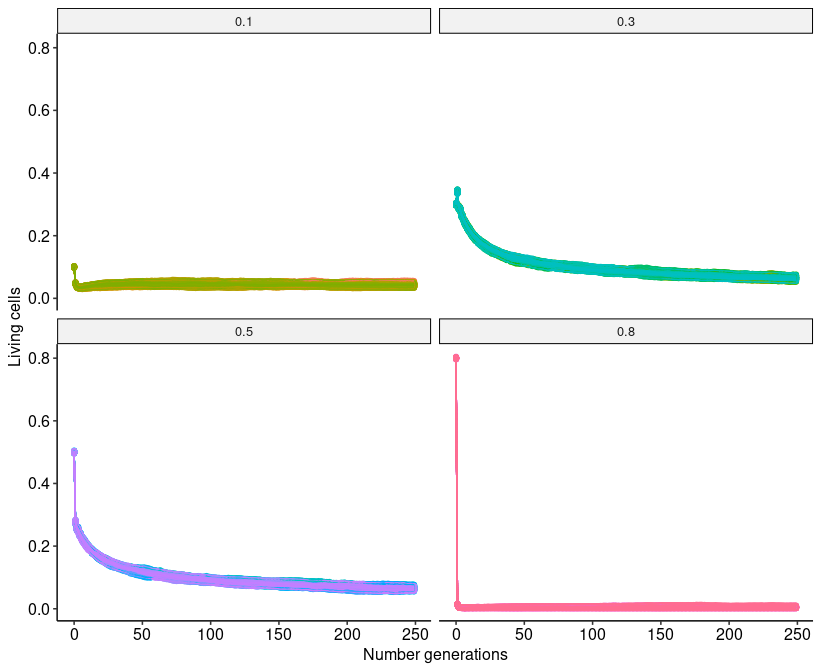I took the day off today to recover from second round of the covid vaccine and spent a little while implementing my own version of Conway’s Game of Life in Python.

Here’s an example of a simulation involving a grid of 500 x 500 cells. When initializing the first generation each cell had a 0.3 probability of starting as a living cell (start-as-living probability). Increasing this value will increase the number of cells the grid is initialized with. I ran the simulation for 100 generations.and with a 0.1 start-as-living probability.I was curious how changing the starting probability of a cell starting the simulation as living would effect the game as it progressed. So I ran 250 generation simulations with start-as-living-cell probabilities of 0.1, 0.3, 0.5 and 0.8. Each start-as-living-cell probability plot is composed of 100 simulations. The generation number is shown on the x-axis and the proportion of living cells is shown on the y.The results where pretty much as one might expect given the game’s rules. Intermediate starting populations level off as the number of generations increase. What was interesting to see was how quickly the number of cells drop if the population exceeds the “carrying capacity” of the grid.